# Delta-Sigma Encoding

February 11, 2020

The delta-sigma encoder is illustrated below. In this topology, the signal enters the loop before the integrator, and the quantization noise follows. In the Laplace transform s-domain, integration is equivalent to multiplication by 1/s.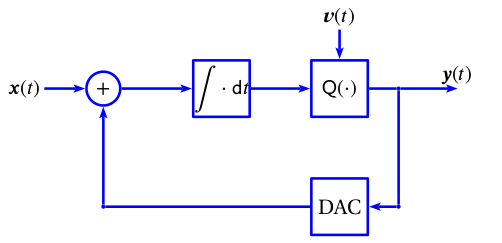Delta-sigma encoder.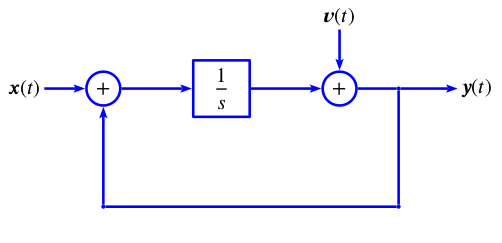Delta-sigma s-domain approximation.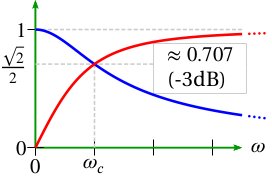Frequency responses

The result of delta-sigma encoding is as follows:

#### Low-Pass Filter

The signal x(t) is low-pass filtered. Why? First assume v(t) = 0. Then:

(1)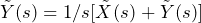(2)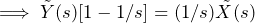(3)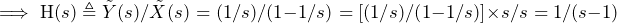Note that in the frequency domain  (at s = ¡ω), this becomes…

(4)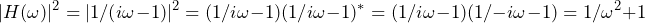… which is a low-pass filter: H(0) = 1 and H(∞) = 0. See the blue line in the “Frequency responses” graph above.

#### High-Pass Filter

The quantization noise v(t) is high-pass filtered. Why? Assume x(t) = 0.  Then:

(5)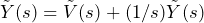(6)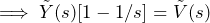(7)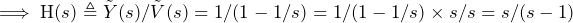Note that in the frequency domain  (at s = ¡ω), this becomes…

(8)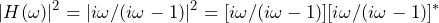(9)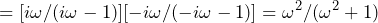… which is a high-pass filter: H(0) = 0 and H(∞) = 1. See the red line in the “Frequency responses” graph above.

Thus, the signal can pass through while the noise is pushed into higher frequency bands. Subsequently, it can be filtered out by a low-pass filter.